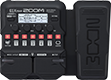# Ed Acoustic 6

Discussion in 'Zoom G1/G1X Four' started by Larry Cesare, Jan 9, 2021.

1. Ed Acoustic 6Device: Zoom G1X Four
Firmware: 2.00

Name on device: Acoustic 6
Optimized for: Phones/Speaker

Effects chain:('cvt_dev' patch): Clean acoustic with Comp, EQ & Reverb

Effect: "Comp" (Dynamics), active - "no"
"Sense" = 2
"Attack" = Slow
"Tone" = 3
"Volume" = 42

Effect: "Gt GEQ 7" (Filter), active - "yes"
"100" = 0.0
"200" = 0.0
"400" = -2.0
"800" = -1.5
"1.6k" = -0.5
"3.2k" = 1.0
"6.4k" = 2.0
"VOL" = 80

Effect: "FD TWNR" (Amp simulator), active - "yes"
"Bass" = 50
"Middle" = 40
"Treble" = 55
"Bright" = OFF
"Gain" = 30
"Volume" = 80
"Depth" = 10
"Speed" = 50

Effect: "FD2x12" (Cabinet), active - "yes"
"MIC" = ON
"D57: D421" = 91
"Hi" = 50
"Lo" = 39

Effect: "Room" (Reverb), active - "yes"
"PreD" = 5
"Decay" = 14
"Mix" = 60
"Tail" = Off

Patch Volume: 100